# Math in Focus Grade 4 Chapter 3 Practice 2 Answer Key Multiplying by a 2-Digit Number

Go through the Math in Focus Grade 4 Workbook Answer Key Chapter 3 Practice 2 Multiplying by a 2-Digit Number to finish your assignments.

## Math in Focus Grade 4 Chapter 3 Practice 2 Answer Key Multiplying by a 2-Digit Number

Example

15 × 10 = 150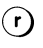63 × 10 = 630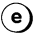Question 1.
5 × 60 = 5 × ___ tens
= ____ tens
= ____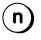5 × 60 = 30.

Explanation:
Given that 5 × 60 which is
5 × 60 = 5 ×6 tens
= 3 tens
= 300.

Question 2.
16 × 20 = 16 × ___ tens
= ___ tens
= ___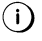16 × 20 = 320.

Explanation:
Given that 16 × 20 which is
16 × 20 = 16 × 2 tens
= 32 tens
= 320.

Question 3.
33 × 40 = 33 × ___ tens
= ___ tens
= ___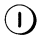33 × 40 = 1320.

Explanation:
Given that 33 × 40 which is
33 × 40 = 33 × 4 tens
= 132 tens
= 1,320.

Question 4.
29 × 30 = 29 × ___ tens
= ___ tens
= ___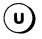29 × 30 = 870.

Explanation:
Given that 29 × 30 which is
29 × 30 = 29 × 3 tens
= 87 tens
= 870.

Question 5.
41 × 60 = 41 × ___ × 10
= ___ × 10
= ___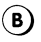41 × 60 = 2,460.

Explanation:
Given that 41 × 60 which is
41 × 60 = 41 × 6 × 10
= 246 × 10
= 2,460.

Question 6.
96 × 40 = 96 × ___ × 4
= ___ × 4
= ___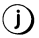96 × 40 = 3,840.

Explanation:
Given that 96 × 40 which is
96 × 40 = 96 × 10 × 4
= 960 × 4
= 3,840.

Question 7.
618 × 50
= 618 × ___ × 10
= ___ × 10
= ___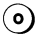618 × 50 = 30,900.

Explanation:
Given that 618 × 50 which is
= 618 × 5 × 10
= 3,090 × 10
= 30,900.

Question 8.
752 × 70
= 752 × ___ × 7
= ___ × 7
= ___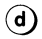752 × 70 = 52,640.

Explanation:
Given that 752 × 70 which is
= 752 × 10 × 7
= 7,520 × 7
= 52,640.

What is the French word that has the same meaning as ‘hello’? Match the letters to the products below to find out.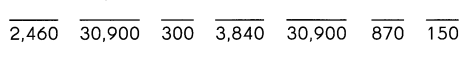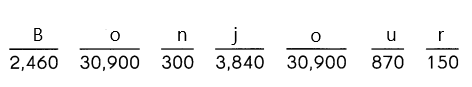Find each product.

Question 9.
42 × 1o = ________
42 × 1o = 420.

Explanation:
The multiplication of 42 × 1o is 420.

Question 10.
786 × 1o = ____
786 × 1o = 7,860.

Explanation:
The multiplication of 786 × 1o is 7,860.

Question 11.
16 × 5 = ____
16 × 50 = ___
16 × 5 = 80,
16 × 50 = 800.

Explanation:
The multiplication of 16 × 5 is 80 and 16 × 50 is 800.

Question 12.
137 × 6 = ___
137 × 60 = ___
137 × 6 = 822,
137 × 60 = 8,220.

Explanation:
The multiplication of 137 × 6 is 822 and 137 × 60 which is 8,220.

Question 13.
23 × 4 = _________
23 × 40 = ___
23 × 4 = 92,
23 × 40 = 920.

Explanation:
The multiplication of 23 × 4 is 92 and 23 × 40 which is 920.

Question 14.
405 × 9 = ___
405 × 90 = ___
405 × 9 = 3,645,
405 × 90 = 36,450.

Explanation:
The multiplication of 405 × 9 is 3,645 and 405 × 90 which is 36,6450.

Find each product.

Question 15.
70 × 800
7 × 8 = ______
7 × 80 = ______
7 × 800 = _______
So, 700 × 800 = ___
7 × 8 = 56,
7 × 80 = 560,
7 × 800 = 5,600,
70 × 800 = 56,000,
700 × 800 = 5,60,000.

Explanation:
The multiplication of 7 × 8 which is 56,so 70 × 800 is 56,000
7 × 80 = 560,
7 × 800 = 5,600,
700 × 800 = 5,60,000.

Question 16.
300 × 90
__________
__________
__________
__________
3 × 9 = 27,
3 × 90 = 270,
3 × 900 = 2,700,
30 × 90 = 2,700,
300 × 90 = 27,000.

Explanation:
The multiplication of 300 × 90 which is 27,000. So
3 × 9 = 27,
3 × 90 = 270,
3 × 900 = 2,700,
30 × 90 = 2,700.

Multiply. Find the missing numbers.

Example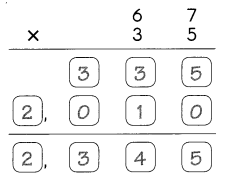Question 17.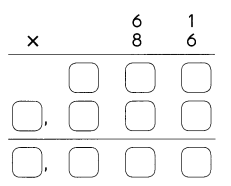61 × 86 = 5,246.

Explanation:
The multiplication of 61 × 86 is 5,246.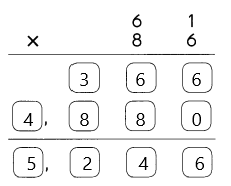Question 18.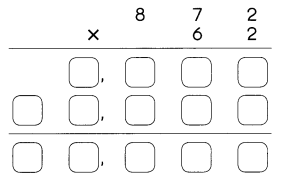872 × 62 = 54,064.

Explanation:
The multiplication of 872 × 62 is 54,064.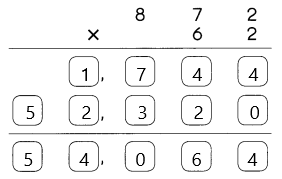Question 19.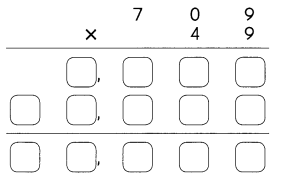709 × 49 = 34,741.

Explanation:
The multiplication of 709 × 49 is 34,741.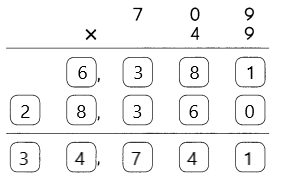Estimate each product. Round each number to its greatest place value.

Example
67 × 35 is about 70 × 40.
70 × 40 = 2,800

Question 20.
61 × 86 is about _____ × ___.
__ × __ = ____
Actual product is 61 × 86 = 5,246.
Estimated product is 60 × 90 = 5,400.

Explanation:
Given that 61 × 86 which is about 60 × 90,
60 × 90 = 5,400.

Question 21.
872 × 62 is about _____ × ____.
__ × __ = ___
Actual product is 872 × 62 = 54,064.
Estimated product is 870 × 60 = 52,200.

Explanation:
Given that 872 × 62 which is about 870 × 60,
870 × 60 = 52,200.

Question 22.
709 × 49 is about ____ × ___.
__ × __ = ___
Actual product is 709 × 49 = 34,741.
Estimated product is 710 × 50 = 35,500.

Explanation:
Given that 709 × 49 which is about 710 × 50,
710 × 50 = 35,500.

Multiply. Then estimate to check that your answers are reasonable. Round each number to its greatest place value.

Example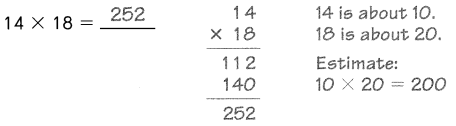252 is close to 200, So, the answer is reasonable.

Question 23.
48 × 21 = ____
Actual product is 48 × 21 = 1,008.
Esstimated product is 50 × 20 = 1,000.

Explanation:
Given that 48 × 21 which is about 50 × 20,
50 × 20 = 1,000.

Question 24.
196 × 34 = ___
Actual product is 196 × 34 = 6,664.
Esstimated product is 200 × 30 = 6,000.

Explanation:
Given that 196 × 34 which is about 200 × 30,
200 × 30 = 6,000.

Question 25.
608 × 73 = ___
Actual product is 608 × 73 = 44,384.
Esstimated product is 610 × 20 = 12,200.

Explanation:
Given that 608 × 73 which is about 610 × 20,
610 × 20 = 12,200.

Question 26.
721 × 54 = ___